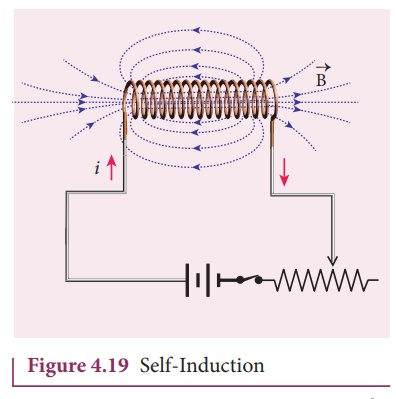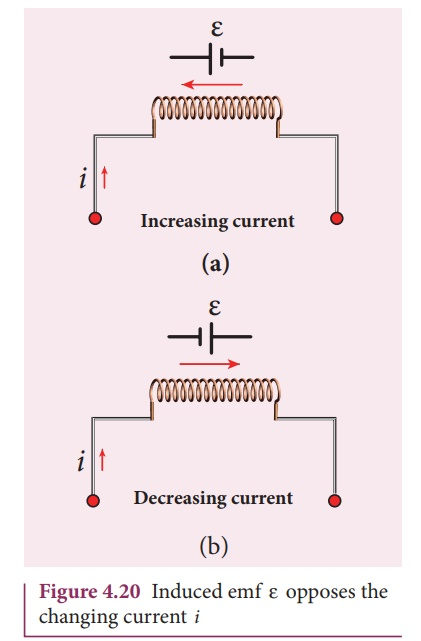Home | | Physics 12th Std | Eddy Currents

# Eddy Currents

Inductor is a device used to store energy in a magnetic field when an electric current flows through it.

SELF-INDUCTION

Introduction

Inductor is a device used to store energy in a magnetic field when an electric current flows through it. The typical examples are coils, solenoids and toroids shown in Figure 4.18.Inductance is the property of inductors to generate emf due to the change in current flowing through that circuit (self-induction) or a change in current through a neighbouring circuit with which it is magnetically linked (mutual induction). We will study about self-induction and mutual induction in the next sections.

## Self-induction

An electric current flowing through a coil will set up a magnetic field around it. Therefore, the magnetic flux of the magnetic field is linked with that coil itself. If this flux is changed by changing the current, an emf is induced in that same coil (Figure 4.19). This phenomenon is known as self-induction. The emf induced is called self-induced emf.Let ╬”B be the magnetic flux linked with each turn of the coil of N turns, then the total flux linked with the coil N╬”B (flux linkage) is proportional to the current i in the coil.The constant of proportionality L is called self-inductance of the coil. It is also referred to as the coefficient of self-induction. If i =1A , then L = N╬”B . Self-inductance or simply inductance of a coil is defined as the flux linkage of the coil when 1A current flows through it.

When the current i changes with time, an emf is induced in it. From FaradayŌĆÖs law of electromagnetic induction, this self-induced emf is given byThe negative sign in the above equation means that the self-induced emf always opposes the change in current with respect to  time.  If  di/dt =1 A sŌłÆ1 ,  then  L =ŌłÆ╬Ą .

Inductance of a coil is also defined as the opposing emf induced in the coil when the rate of change of current through the coil is 1 AŌĆåsŌłÆ1.

## Unit of inductance

Inductance is a scalar and its unit is Wb A-1 or V s A-1 . It is also measured in henry (H).

H = 1 W b AŌłÆ 1 =1 V s AŌłÆ1

The dimensional formula of inductance is M L2  T - 2 A-2 .

If i = 1 A and N ╬”B =1 Wb turns, then =1H .

Therefore, the inductance of the coil is said to be one henry if a current of 1ŌĆåA produces unit flux linkage in the coil.

If di/dt = 1 AsŌłÆ 1  and ╬Ą =ŌłÆ1 V , then L H =1 .

Therefore, the inductance of the coil is one henry if a current changing at the rate of 1 AŌĆåsŌłÆ1 induces an opposing emf of 1ŌĆåV in it.

## Physical significance of inductance

We have learnt about inertia in XI standard. In translational motion, mass is a measure of inertia; in the same way, for rotational motion, moment of inertia is a measure of rotational inertia (Refer sections 3.2.1 and 5.4 of XI physics text book). Generally, inertia means opposition to change its state.

The inductance plays the same role in a circuit as mass and moment of inertia play in mechanical motion. When a circuit is switched on, the increasing current induces an emf which opposes the growth of current in a circuit (Figure 4.20(a)). Likewise, when circuit is broken, the decreasing current induces an emf in the reverse direction. This emf now opposes the decay of current (Figure 4.20(b)).

Thus, inductance of the coil opposes any change in current and tries to maintain the original state.Tags : Introduction, Definition, Formula, Physical significance | Electromagnetic Induction , 12th Physics : Electromagnetic Induction and Alternating Current
Study Material, Lecturing Notes, Assignment, Reference, Wiki description explanation, brief detail
12th Physics : Electromagnetic Induction and Alternating Current : Eddy Currents | Introduction, Definition, Formula, Physical significance | Electromagnetic Induction